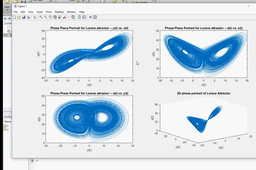# [VIDEO] Matlab Tutorial: Integrate Ordinary Differential Equations ODE’s (Lorenz Equation)How to integrate Ordinary Differential Equations ODE’s (Lorenz Equation) with the aid of Matlab?
This tutorial shows how to do gather a solution!